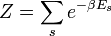# Statistical mechanics - Partition function of a system of N particles

## Homework Statement

Imagine a system with N distinguishable particles. Each particle may be in two states of energy: -ε and +ε.

Find the the partition function of the system

## Homework Equations## The Attempt at a Solution

I know that I have to find the partition function for a single function, Z, and my final result will be ZN. Now, I'll say that:

(Where it says ε it's meant to be ε(r) )

Z = Ʃr exp(-β(ε - ε) ) = Ʃr exp(-βε) * exp(βε) =
= Ʃr exp(-βε) * Ʃr exp(βε)

I'm sure this is incorrect. It doesn't make sense in my head.. E(r) is the energy associated with each microstate, therefore saying that E(r) = ε(r) - ε(r) can't make any sense! I know that the result is:

Z = ( exp(βε) + exp(-βε) )N

I have no idea how to get there tho. How did it became a sum? How do I get rid of the summatories?

Any help will be appreciated!
Thanks.

The partition function is a summation over states. You simply are using the summation wrong. It is not a summation over the energy levels of within the exponent. It is a summation over e(-Es/T).

Look at any example problem in a thermo book for a 2-state system

8ikmAm I not summing over the expoent of the energy of each microstate?

Look at any example problem in a thermo book for a 2-state system

Do you know any good statistical mechanics book you'd advise me reading?

EDIT:
Is it a summation over all the states of energy instead of the energies of each microstate? Because then the solution would make sense!

Last edited:
Am I not summing over the expoent of the energy of each microstate?

Do you know any good statistical mechanics book you'd advise me reading?

No. You are summing over the exponential function raised to the -Es/T.

A good book is Thermal Physics by Kittel + Kroemer

Where Es is the energy of the s-th state